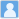• ### Radial variations of outward and inward Alfvnic fluctuations based on Ulysses observations

分类： 地球科学 >> 空间物理学 提交时间： 2017-03-31Yang, L. Lee, L. C. Li, J. P. Luo, Q. Y. Kuo, C. L. Shi, J. K. Wu, D. J.

摘要：Ulysses magnetic and plasma data are used to study hourly-scale Alfv{\'e}nic fluctuations in the solar polar wind. The calculated energy ratio $R^2_{v_A}$(cal) of inward to outward Alfv{\'e}n waves is obtained from the observed Wal{\'e}n slope through an analytical expression, and the observed $R^2_{v_A}$(obs) is based on a direct decomposition of original Alfv{\'e}nic fluctuations into outward- and inward-propagating Alfv{\'e}n waves. The radial variation of $R^2_{v_A}$(cal) shows a monotonically increasing trend with heliocentric distance $r$, implying the increasing local generation or contribution of inward Alfv{\'e}n waves. The contribution is also shown by the radial increase in the occurrence of dominant inward fluctuations. We further pointed out a higher occurrence ($\sim 83\%$ of a day in average) of dominant outward Alfv{\'e}nic fluctuations in the solar wind than previously estimated. Since $R^2_{v_A}$(cal) is more accurate than $R^2_{v_A}$(obs) in the measurement of the energy ratio for dominant outward fluctuations, the values of $R^2_{v_A}$(cal) in our results are likely more realistic in the solar wind than previously estimated and than $R^2_{v_A}$(obs) in our results. The duration ratio $R_T$ of dominant inward to all Alfv{\'e}nic fluctuations increases monotonically with $r$, and is about two or more times that from {\it Voyager 2} observations at $r \geq 4$~AU. Finally, from the variation trend in our results, a higher (lower) occurrence rate is expected at $r < 1$~AU ($r > 4$~AU) for dominant outward Alfv{\'e}nic fluctuations, and opposite variations are expected for dominant inward fluctuations. Simultaneously, $R^2_{v_A}$(cal) and $R_T$ will be expected to be smaller at $r < 1$~AU and larger at $r > 4$~AU. These results reveal new qualitative and quantitative features of Alfv{\'e}nic fluctuations therein compared with previous studies and put constraints on modelling the variation of solar wind fluctuations.

同行评议状态:待评议